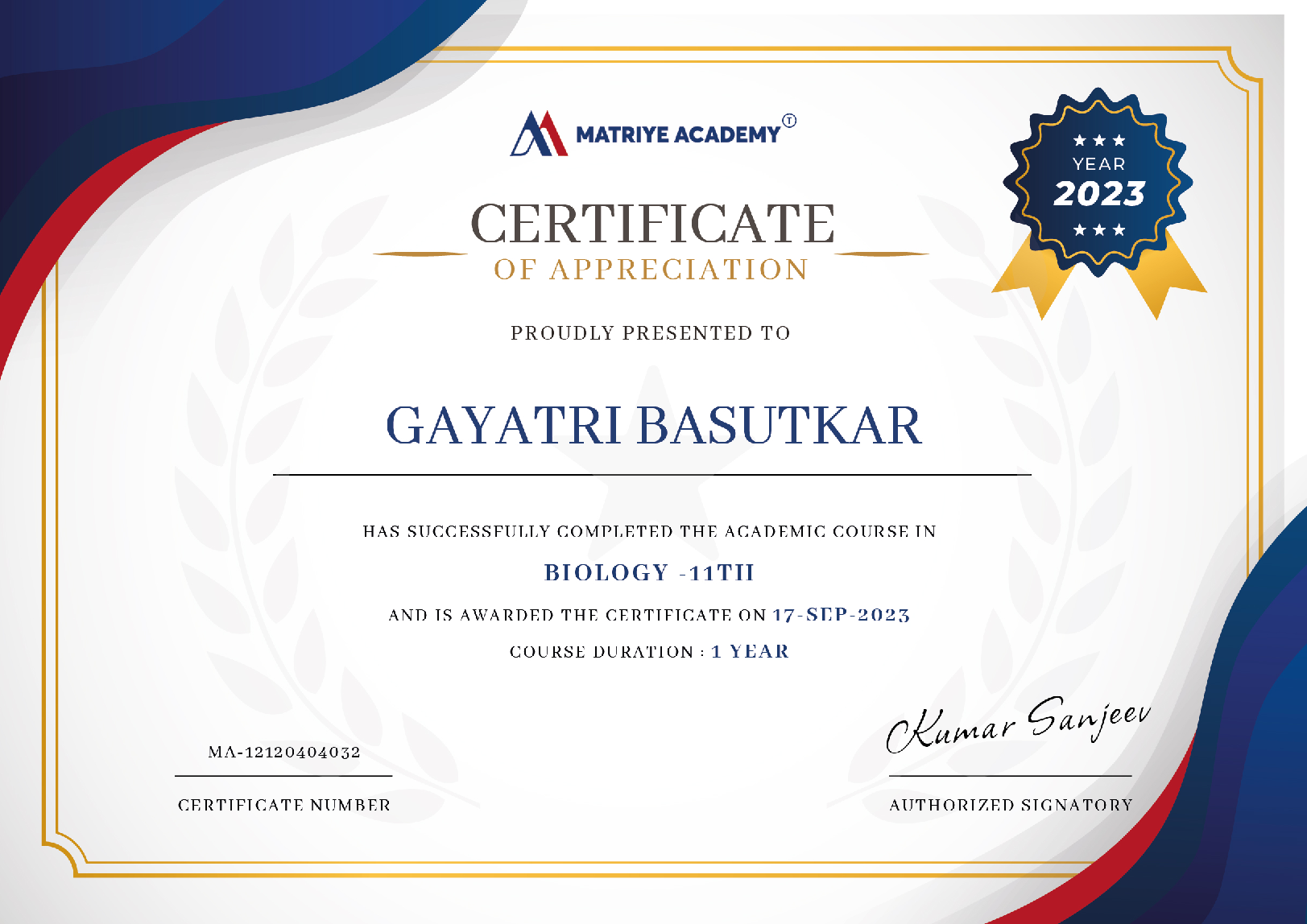# Math's

CBSE 8

Class 8 Math is the first platform for students to study essential ideas and their applications.##### Runali Deb Roy

Last Update : 07 Feb 2023

(4)

## Course Overview

Class 8 Mathematics material elevates topics such as rational numbers, data processing, algebra, mensuration, exponents, and powers by connecting them to their initial components covered in previous classes. This grade introduces concepts such as linear equations, squares and square roots, cubes and cube roots, profit and loss, percentage, interest, and direct and inverse proportions.

The Matriye Academy Class 8 curriculum Math assists pupils in comprehending mathematical principles and then applying that understanding to real-world circumstances. The goal is to help pupils improve their decision-making abilities, accuracy, analytical thinking, and reasoning. Matriye Academy's many learning assets contribute to the achievement of the stated goal.

### What You'll Learn

• Learn, practice, and advance their knowledge at the comfort and safety of their homes.
• Explore and explain the absolute values of numbers.
• Students will learn how to simplify expressions and the corresponding forms of big and small integers in scientific and standard notation.

16 Lessons 45m

### CBSE Curriculum, Class 8th Math

India, CBSE Curriculum, Class 8th Math. The CBSE Pattern Class 8 mathematics curriculum will educate students about real numbers, rational numbers, and the approximation of irrational values to rational numbers. Students in class 8 math are required to understand the four basic characteristics of numbers: commutative, associative, closure, and distributive. Following that, students will study basic mathematics, with an emphasis on percentage, percentage comparison, and compound interest approaches. As students assimilate fundamental algebra, the focus shifts to algebra-I subjects, such as expressions and equations, division and multiplication of expressions, exponents, square roots and cube roots, data processing and analysis, and visualizing 3-D objects.

### NCERT Solutions for Class 8 Maths by Chapter

Class 8th NCERT Solutions Students will benefit much from the math presented here. They may use these NCERT Solutions for Class 8 as a reference for practicing problems and developing improved abilities, as well as efficiently preparing for the examinations. Exercises are provided at the conclusion of each chapter to allow students to put what they've learned into practice.

### Class 8 NCERT Solutions for Rational Numbers

Students will study about independent, associative, and closure properties of real numbers, integers, whole numbers, rational numbers, and natural numbers in this chapter. The function of zero and one, multiplication over addition, depiction of rational numbers on the number line, and searching for rational numbers between two rational numbers are all covered in this chapter.## 1

##### Number System
5
5 ratings 4 reviews

1. Rational Numbers
2. Powers
3. Squares, Square roots, Cubes, Cube roots
4. Playing with numbers

## 2

##### Algebra
5
4 ratings 3 reviews

1. Algebraic Expressions

### Course Instructor##### Shruti Koli
(4)
25 Tutorials 100 Students

There are so many courses, so many professors, and so many high-quality study materials available on it. You may also administer quizzes, which are quite useful for improving your time management abilities. I enjoy taking quizzes since it boosts my confidence as well as my knowledge##### Ravikant Sharma
(4)
25 Tutorials 100 Students

Matriye Academy is one of the greatest platforms for studying and learning the chapters in a clever way, with animations and enjoyment. I don't think any other platform, other than Byjus, provides such a broad experience.

### Start Learning Today

###### Financial aid available
• Taught by top companies and universities
• Affordable programs
• Apply your skills with hands-on projects
• Learn on your own schedule